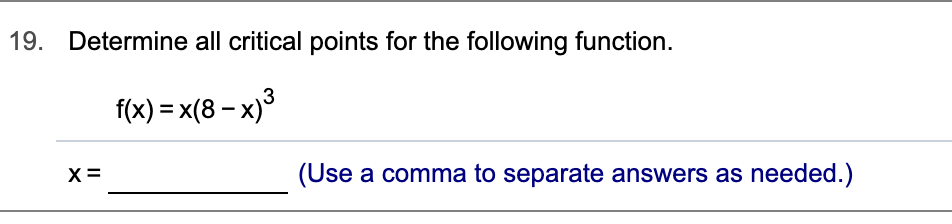# Determine all critical points for the following function19.f(x) x(8-x)3_(Use a comma to separate answers as needed.)х3

Question
58 views

Can you help me step by step?help_outlineImage TranscriptioncloseDetermine all critical points for the following function 19. f(x) x(8-x)3 _ (Use a comma to separate answers as needed.) х3 fullscreen
check_circle

Step 1

Find the critical points of the given function:

Step 2

To determine the critical points of the given function, find the first derivative and then set the first derivative equal to 0.

Now expand the function first.

Step 3

Firstly find the first derivative of t...

### Want to see the full answer?

See Solution

#### Want to see this answer and more?

Solutions are written by subject experts who are available 24/7. Questions are typically answered within 1 hour.*

See Solution
*Response times may vary by subject and question.
Tagged in

### Derivative